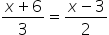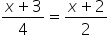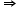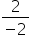Maths-
General
Easy

Question

# Solve the equationHint:

## The correct answer is: x = 21

### ○ Given:Equation:○ Step 1:○ Cross multiply:(x + 3)  2 = (x + 2)  42x + 6 = 4x + 8○ Step 2:Group like terms:2x - 4x = 8 - 6- 2x = 2x =x = -1 Final Answer: x = -1#### With Turito Foundation.#### Get an Expert Advice From Turito.